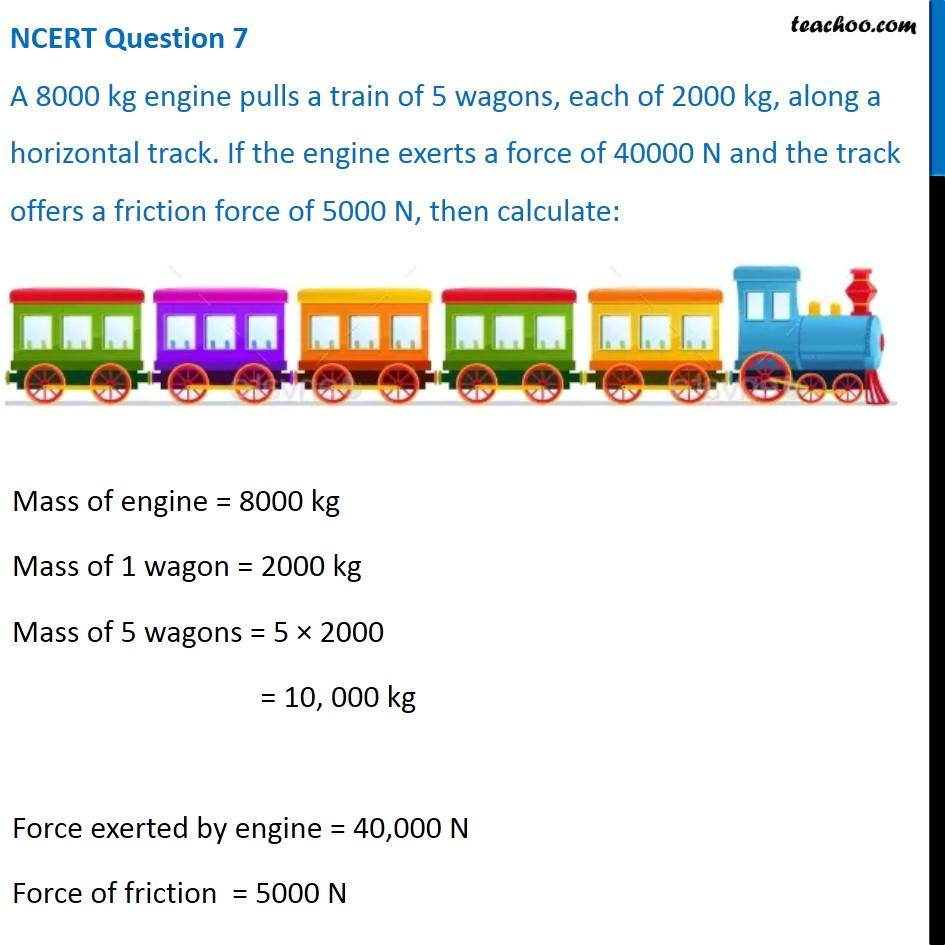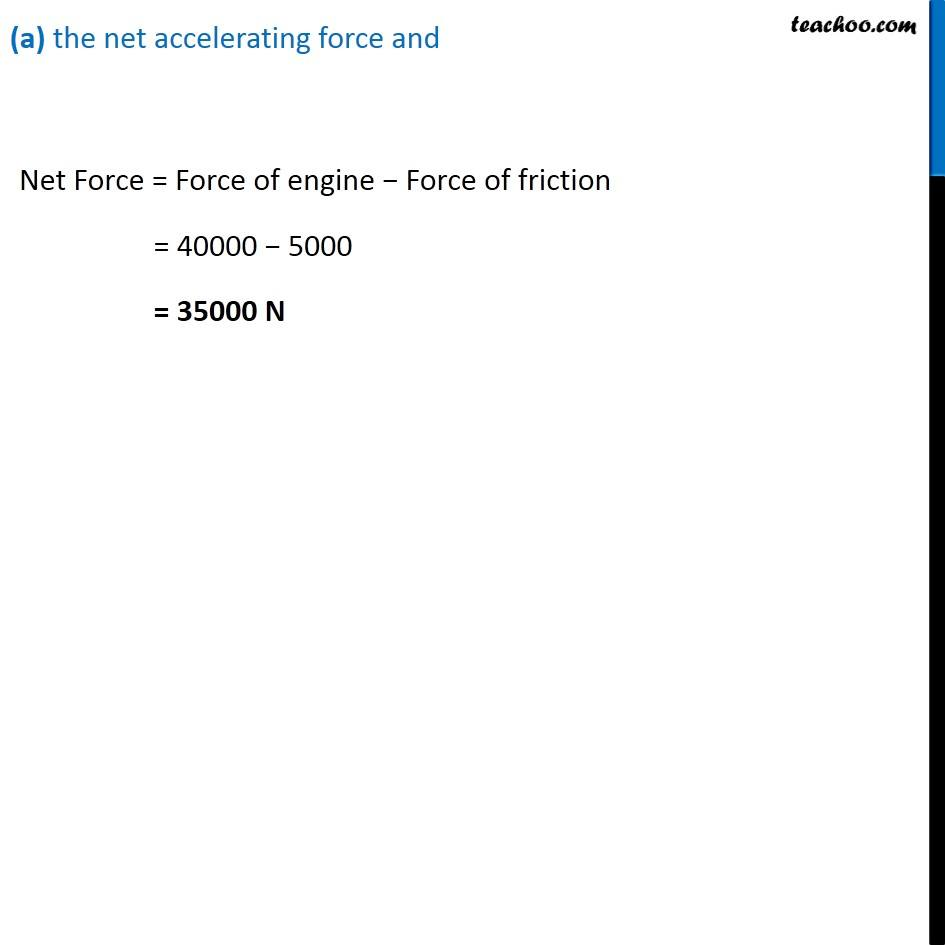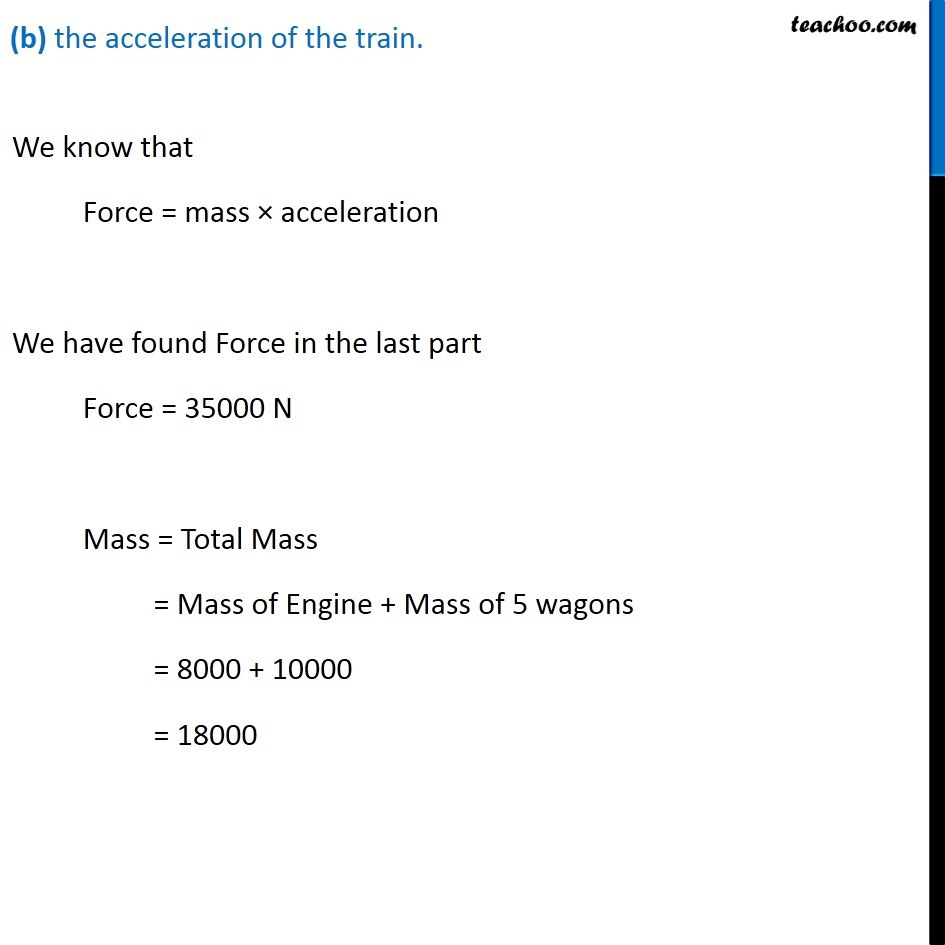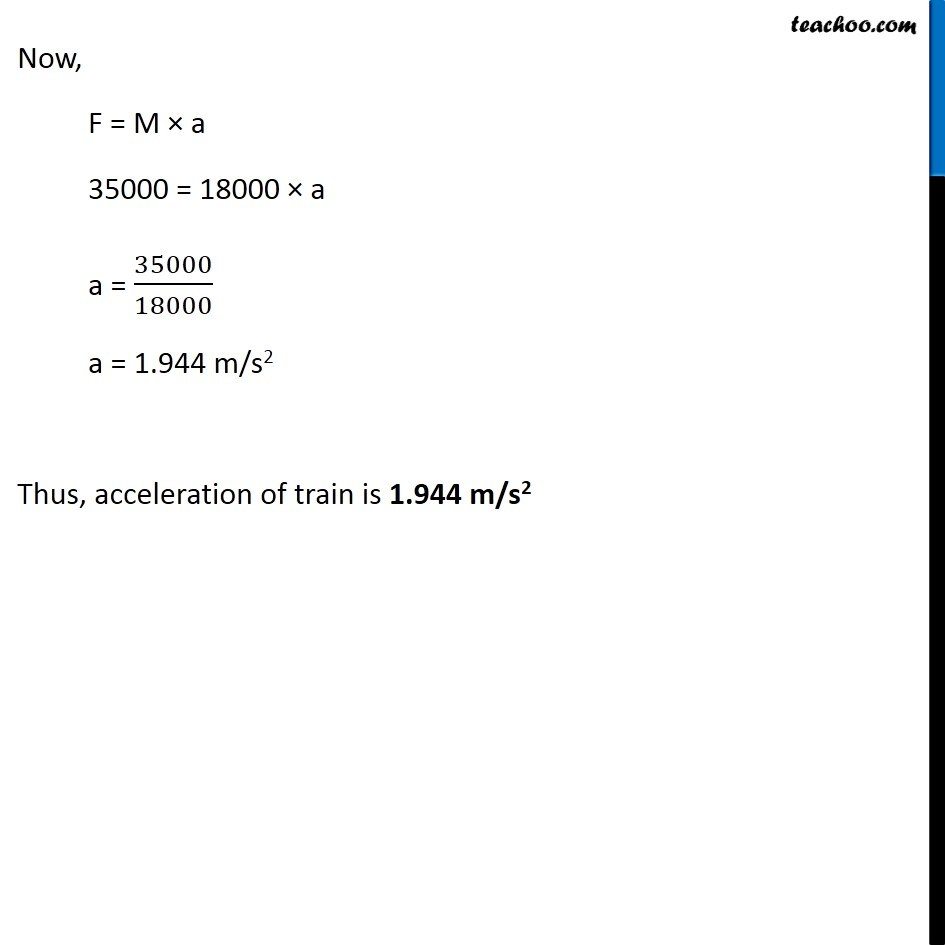NCERT Questions

Class 9
Chapter 9 Class 9 - Force and Laws Of MotionGet live Maths 1-on-1 Classs - Class 6 to 12

### Transcript

NCERT Question 7 A 8000 kg engine pulls a train of 5 wagons, each of 2000 kg, along a horizontal track. If the engine exerts a force of 40000 N and the track offers a friction force of 5000 N, then calculate: Mass of engine = 8000 kg Mass of 1 wagon = 2000 kg Mass of 5 wagons = 5 × 2000 = 10, 000 kg Force exerted by engine = 40,000 N Force of friction = 5000 N (a) the net accelerating force and Net Force = Force of engine − Force of friction = 40000 − 5000 = 35000 N (b) the acceleration of the train. We know that Force = mass × acceleration We have found Force in the last part Force = 35000 N Mass = Total Mass = Mass of Engine + Mass of 5 wagons = 8000 + 10000 = 18000 Now, F = M × a 35000 = 18000 × a a = 35000/18000 a = 1.944 m/s2 Thus, acceleration of train is 1.944 m/s2